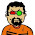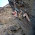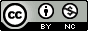## Monday, January 11, 2010

### ggplot2 Tutorial: Scatterplots in a Series of Small Multiples

It took several months after learning about ggplot2 before I gave it a try myself.  I was apprehensive about learning a new graphics system with a new set of commands.  Thing is, if you've ever used plot() in R, you already know how to use much of the functionality in ggplot2!  In this tutorial I want to show you just how easy it is to start producing high-quality graphics using ggplot2.  I'll also introduce the concept of a series of small multiples, and show you how ggplot2 makes it dead simple to produce these kinds of graphics yourself.

Getting started:

First of all, if you've never used ggplot2 before, you'll need to download and install it.  Fire up R, and type this command, exactly as is, with the quotes:

install.packages("ggplot2")

Pick a mirror that's close to you, and install.  Once you do this, or if you've already installed ggplot2 in the past, load the ggplot2 library (no quotes this time).  You'll have to do this every time you start a new R session.

library(ggplot2)

Now we need some data.  Rather than making up our own data, let's use the "diamonds" dataset that comes packaged with ggplot2. Type this command:

data(diamonds)

This dataset contains ~50,000 entries.  Each row is an individual diamond, and some of the variables of interest include the weight of the diamond in carats, color, clarity, and its price. We can get a very good picture of the relationship between these variables using some fairly simple plotting commands.  First let's take a look at the first few rows of the dataset using the head() function.

Here's what the first few rows of the relevant columns look like:

```   carat price color clarity
1   0.23   326     E     SI2
2   0.21   326     E     SI1
3   0.23   327     E     VS1
4   0.29   334     I     VS2
5   0.31   335     J     SI2
6   0.24   336     J    VVS2
7   0.24   336     I    VVS1
8   0.26   337     H     SI1
9   0.22   337     E     VS2
10  0.23   338     H     VS1

```

The variables carat and price are both continuous variables, while color and clarity are discrete, or in R lingo, factor variables.  Wikipedia has nice charts describing what these codes mean for a diamond's color and clarity.

First, let's take a look at the distribution of price using a histogram.

Using base graphics:

with(diamonds, hist(price))

And using the qplot function ggplot2:

qplot(price, data=diamonds)

You may have gotten a warning that you didn't specify the bin width.  Hadley Wickham, ggplot2's creator, seems like a nice guy.  He encourages you to specify how wide you want the bins, but even if you don't, ggplot2 will still make your plot using sensible defaults.  Let's specify the binwidth ourselves this time:

qplot(price, data=diamonds, binwidth=1000)

That plot looks a lot like the defaults using base graphics.  Now let's take a look at the relationship between the price of a diamond and its weight in carats.

Using base graphics:

with(diamonds, plot(carat,price))

And using ggplot2:

qplot(carat, price, data=diamonds)

Notice something about the ggplot2 syntax here. Using base R graphics there are different commands for scatterplots and histograms. But in ggplot2, if you specify a single continuous variable to the qplot command, you'll get a histogram. If you specify two continuous variables to the same qplot command, you get a scatterplot. The bars in a histogram and the points on a scatterplot are called "geoms" in ggplot2 lingo.  You can specify exactly which geom you want to use, but since Hadley's such a nice guy, ggplot2 will often guess which type of "geom" you want to use based on the data type you give it.  Let's say you wanted to look at the distribution of price using the kernel density estimation, rather than a histogram.  The command is very similar to the command you used to get a histogram, you just specify "density" as the geom, instead of leaving this option out:

qplot(price, data=diamonds, geom="density")

As you can see, using different types of "geoms" with different data types allows you to break many graphical conventions. This is usually a bad thing, but this also allows you to be creative and design your own way to display data.  Anyhow, let's get back to scatterplots.

Let's say you want to add labels and a main title to your plot using xlab, ylab, and main.  If you know how to do this using base graphics, then you already know how to do this using ggplot2.

Using base graphics:
with(diamonds, plot(carat,price, xlab="Weight in Carats", ylab="Price in USD", main="Diamonds are expensive!"))

And using ggplot2:
qplot(carat, price, data=diamonds, xlab="Weight in Carats", ylab="Price in USD", main="Diamonds are expensive!")

All we've done so far is to examine the relationship between carat and price.  As one would expect, the price increases sharply as the size of the diamond increases.  But what about the other variables here, color and clarity? Specifically, does color or clarity modify the effect of carat on price? In other words, is there an interaction between carat and either of these variables?

To visualize this graphically, we could plot price vs carat for each level of one of the other two vactors, color or clarity. In ggplot2 terminology, we could facet the plot by one of these factor variables. Let's look at color first. The facets option does just this.  The syntax "color~." is saying that we want plots of price vs carat by color, where color is on the rows.  If you wanted separate facets beside each other in columns, the syntax would look like ".~color".

qplot(carat, price, data=diamonds, facets=color~.)

This plot reveals something important about the relationship between these three variables.  As the letters go from D to J, the diamond becomes more and more yellow.  This plot shows us that as the diamond's color becomes more white, an increase in carat causes a faster increase in price.  In other words, the color of the diamond modifies the effect of carat.

We can formally test in linear regression using the lm (linear model) command.  Note that I'm treating color now as a continuous (numeric) variable.  The output shows us that the interaction is significant at p<2e-16.

```fit1=lm(price~carat*as.numeric(color), data=diamonds)
summary(fit1)

Call:
lm(formula = price ~ carat * as.numeric(color), data = diamonds)

Residuals:
Min        1Q    Median        3Q       Max
-15445.88   -813.27    -20.89    634.69  11740.29

Coefficients:
Estimate Std. Error t value Pr(>|t|)
(Intercept)             -2532.529     29.871 -84.782   <2e-16 ***
carat                    9276.490     35.789 259.202   <2e-16 ***
as.numeric(color)          -3.227      7.445  -0.433    0.665
carat:as.numeric(color)  -298.153      7.777 -38.340   <2e-16 ***
---
Signif. codes:  0 ‘***’ 0.001 ‘**’ 0.01 ‘*’ 0.05 ‘.’ 0.1 ‘ ’ 1

Residual standard error: 1476 on 53936 degrees of freedom
Multiple R-squared: 0.8631,     Adjusted R-squared: 0.8631
F-statistic: 1.134e+05 on 3 and 53936 DF,  p-value: < 2.2e-16
```

You can also do the same thing for clarity (omitting the statistical analysis):

qplot(carat, price, data=diamonds, facets=clarity~.)

As you go down the panels the diamond gets more and more clear.  The numbers beside "S" (small) and "VS" (very small) describe the size of the "inclusions", or internal imperfections, in the stones. "IF" means internally flawless, a rare find.  As you'd expect, as the diamond becomes more and more flawless, the price per carat dramatically increases.

Finally, as you'd imagine, you can facet by both color and clarity, one on the columns and one on the rows.  You'll have to click on the image below to see it full-screen.

qplot(carat, price, data=diamonds, facets=clarity~color)

The bottom left panel shows price vs carat for very white, internally flawless diamonds.  Notice how few observations are in this cell compared to others.  These are extremely rare and expensive stones.  Compare the relationship here with the relationship in the upper right facet, where you have the yellowest, and dirtiest diamonds. You can statistically test this one as well, and you'll find that there's a highly significant (p<1e-16) three-way interaction between carat, color, and clarity, that influences the price of the diamond.

This last plot above brings me to the topic in the title, "small multiples." This term, popularized by Edward Tufte, refers to visually displaying relationships in data using the same type of figure over and over again.  The idea here is that you teach the reader once how to interpret each individual component.  Once the reader is oriented to a single component, you can show small multiples of the same figure over and over again to display how relationships change based on certain factors, without requiring the reader to figure out how to decode a new graphic every time. The fact that the figure above distills 215,760 data points across 4 dimensions into a graphic that you can interpret within seconds proves that using small multiples can be a highly effective way to display large, high-dimensional data. Do an image search for small multiples to see many more examples.  Tufte is cited in chapter 1 of the ggplot2 book, and it's very adept at displaying complex relationships in big data in an intuitive and effective manner.

I only used this diamonds dataset because it's free and readily available once you install ggplot2. There are so many ways you can use this technique for data visualization in genetics, where interactions between variables and their influence on traits are difficult to ignore.  I hope I've convinced you that using ggplot2 is no harder than base graphics, and makes high-dimensional plotting very easy. If you've found this tutorial useful, let me know in the comments, and I'll try to post a few more like this later on.

1.Very useful tutorial, I enjoyed it very much - thank you :)

2.Wonderful tutorial, thank you!
allattention

3.Great tutorial using ggplot2...my new favorite R package

4.This help so much. Thank you. My new favorite blog.

5.Thanks for the excellant introduction. It would be great if you did more in this series.

6.Very good! I'm glad I found this blog.

7.Good post. Can you mix faceted plots and viewports.

That is if I make two or more plots with facets can I arrange them on a grid.

I have been trying using the methods in chapter 8 of the book but can't get it to work.

regards

8.What a tutorial!!!!!!!!!!!!!!!!!!
Amazin>>>>>
Thanx so much

9.very helpful - i'm a statistician learning how to use ggplot and this helped me. thanks!

10.Thanks!

11.Great introduction - thanks!

12.Thank you so much for this. This is exactly what I needed to hit the ground running with R/ggplot2!

13.14.15.Thanks much for this. New to R and the book doesn't even mention that you need to FRIST use the "Library" and "Data" command to get rolling,

16.Quick, clear, and concise. Very helpful. Thanks!

17.Please can you explain why you convert color to numeric in the modelling? It is not necessary, is it for some reason?

18.Good Tutorial.

19.Good post, but your images are not present anymore..!!

20.Thanks, working on that! I hosted them on a Vanderbilt server when I worked there, but they seemed to have deleted my images when I left. I'm in touch with the IT folks now.

21.Finally, regenerated some of the images.

22.This is HELPED ME A LOT AND ANSWERED LOTS OF QUESTIONS. Thank you for posting this. Very clear and to the point.

23.Hi, I try to get dataset(diamonds)
I run:
> library(ggplot2)
Error in loadNamespace(i, c(lib.loc, .libPaths())) :
there is no package called ‘plyr’
Error: package/namespace load failed for ‘ggplot2’
> data(diamonds)
Warning message:
>

How to solve this ? Thanks

1.looks like you need to manually install plyr:

install.packages("plyr")

Load ggplot2, the data(diamonds) should work.

24.Thanks so much for this! Great intro to ggplot2, and excellent overview of small multiples.

25.Thanks a lot. I'm new to R and quietly terrified of it but this helped calm my nerves.

26.could you please explain how to statistically analyse the final graph with the two continuos data and two factors? to get the (p<1e-16) three-way interaction between carat, color, and clarity, that influences the price of the diamond.